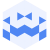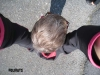Am 23. May. 2023 | 19:45 Uhr

# Billy Roisz | Tresor Linz I May 23 2023 | canceled but not canceledCreated at 29. May. 2023

141 Ansichten
##### by Tresor Linz

Tresor Linz - recorded on May 23 2023

<<<<<<<<<<<<<<<<<<<<<<<<<<<<<<<<<<<<<<<<<<<<<<<<<<<<<<<<<

Billy Roisz BAJO

<<<<<<<<<<<<<<<<<<<<<<<<<<<<<<<<<<<<<<<<<<<<<<<<<<<<<<<<<

Billy Roisz has been a driving force in the experimental music scene for many years. She is widely known and has earned critical acclaim for her artistic work, which ranges from cinematic (her films are regularly screened at major film-festivals such as Berlinale-Shorts or the IFF Rotterdam) to pure audio-works, as she presents with this new album. Her last solo-release »Walking The Monkey« dates back to 2012 (Editions Mego) and now she unveils her successor: »BAJO« A sonic exploration of double bass from a nosey and refreshingly innocent point of view. Roisz investigates the concept of going low - not only in terms of frequency, but also in a metaphysical sense; expecting the album to be a droning low-end scenario is completely wrong. Billy Roisz manages to compose music that indeed feels as if you are under an obscure yet fascinating viscose or earthy substance, that evokes the heavy need inside the listener to dig even deeper, making »BAJO« a journey into the depths of sound par excellence. Roisz seamlessly blends the acoustic signals of the double bass with the modulation of electronic devices, deliberately adding only the sound of one single sonic entity, created by a cymbal that belonged to her longtime stage partner Silvia Fässler of the duo »Skylla«. Almost as a signature-move, Roisz uses errors and surprise-encounters as a pathfinder to compose multifaceted pieces full of intriguing sonic territories and unexpected associations. Indeed, »BAJO« takes the listeners deep down the burrow - where one experiences what might be considered as Billy Roisz’s very own ritual music.

<<<<<<<<<<<<<<<<<<<<<<<<<<<<<<<<<<<<<<<<<<<<<<<<<<<<<<<<<
Tresor Linz
<<<<<<<<<<<<<<<<<<<<<<<<<<<<<<<<<<<<<<<<<<<<<<<<<<<<<<<<<

Raum für Klangkunst und künstlerische Experimente.

Space for sound art and artistic experiments.

##### Share & Embed
Embed this Video##### by gabi
6 months 1 week ago

Ganz wunderbar. Vielen Dank Stefan Tiefengraber für die Tresor - Konzerte!#### Tresor Linz

47 Videos, 2 Members